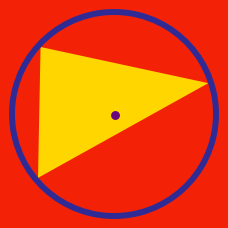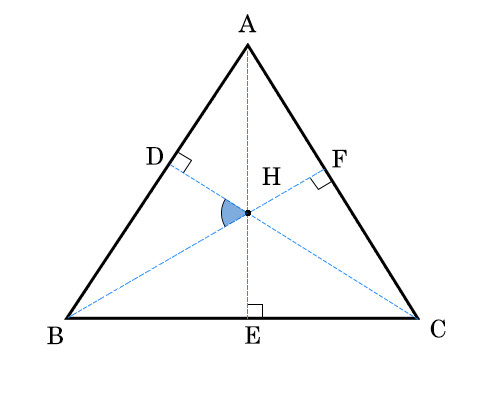Geometry

# Triangles - Orthocenter

The orthocenter is the intersection of which 3 lines in a triangle?

Where is the orthocenter of an obtuse triangle?

Where is the orthocenter of a right triangle?

Does every triangle have its orthocenter?Point $H$ is the orthocenter of $\triangle{ABC}$. If $\angle{A}=59^\circ$, $\angle{B}=60^\circ$, and $\angle{C}=61^\circ$, what is the measure of $\angle{BHD}$?

×